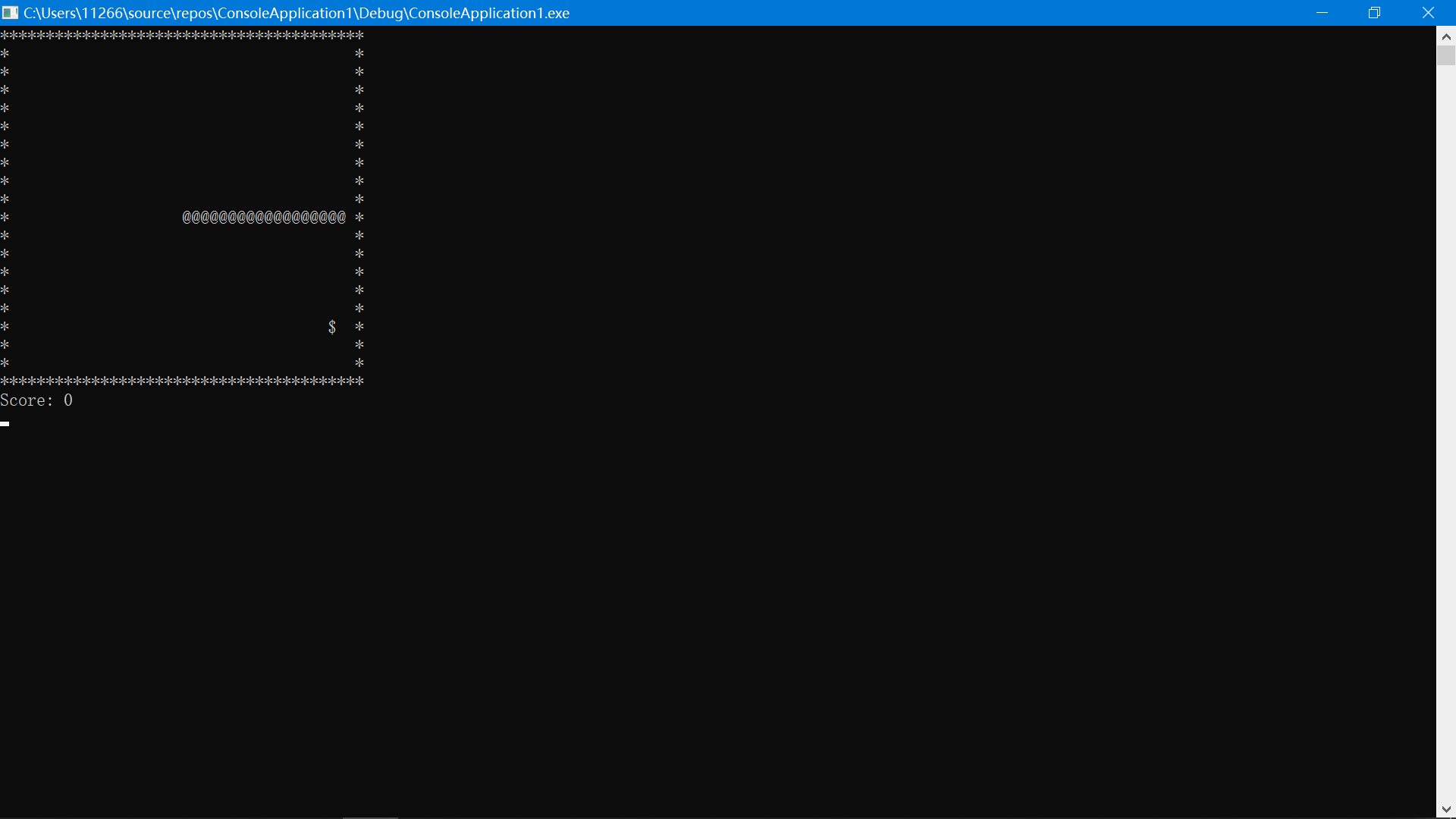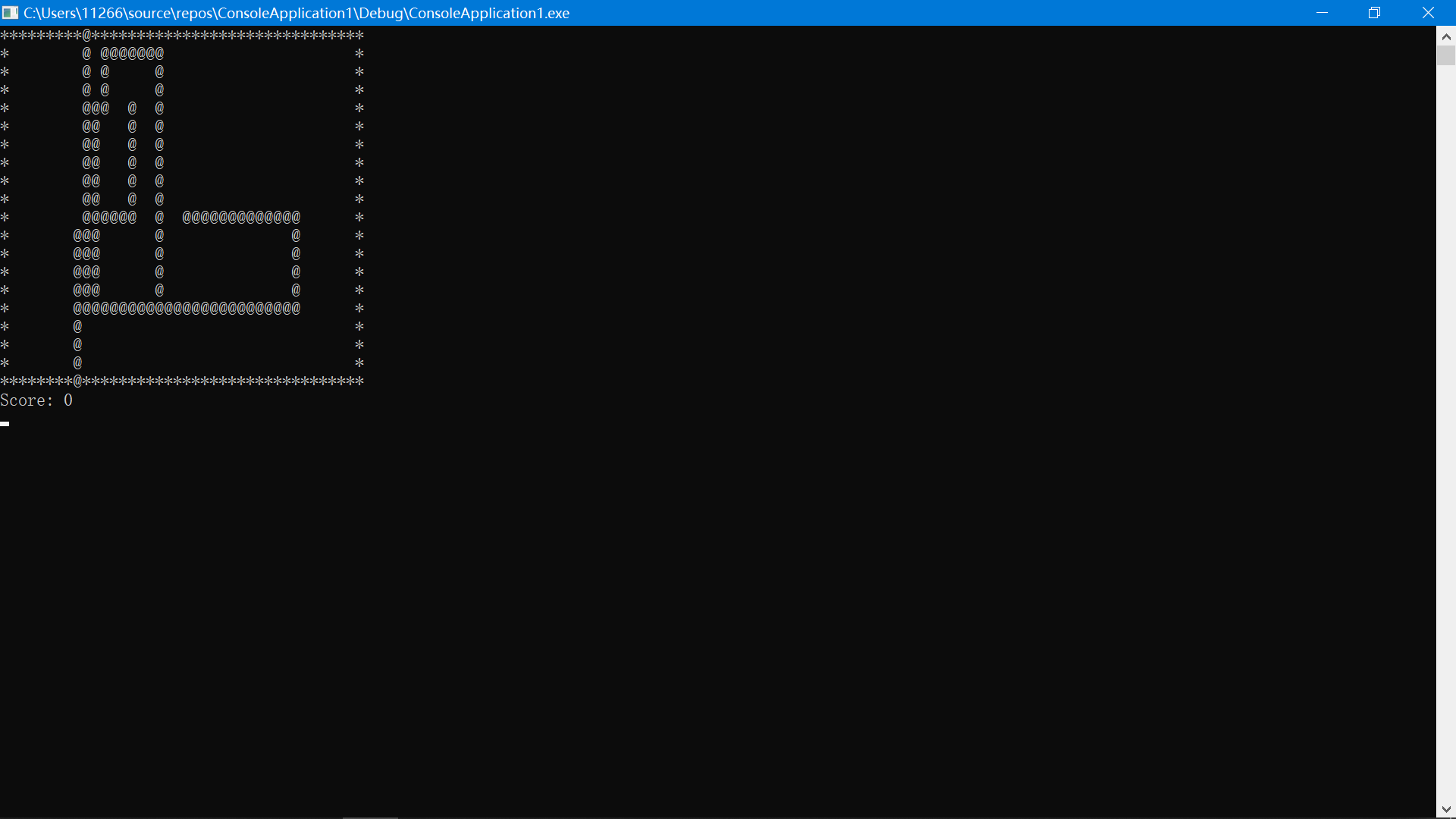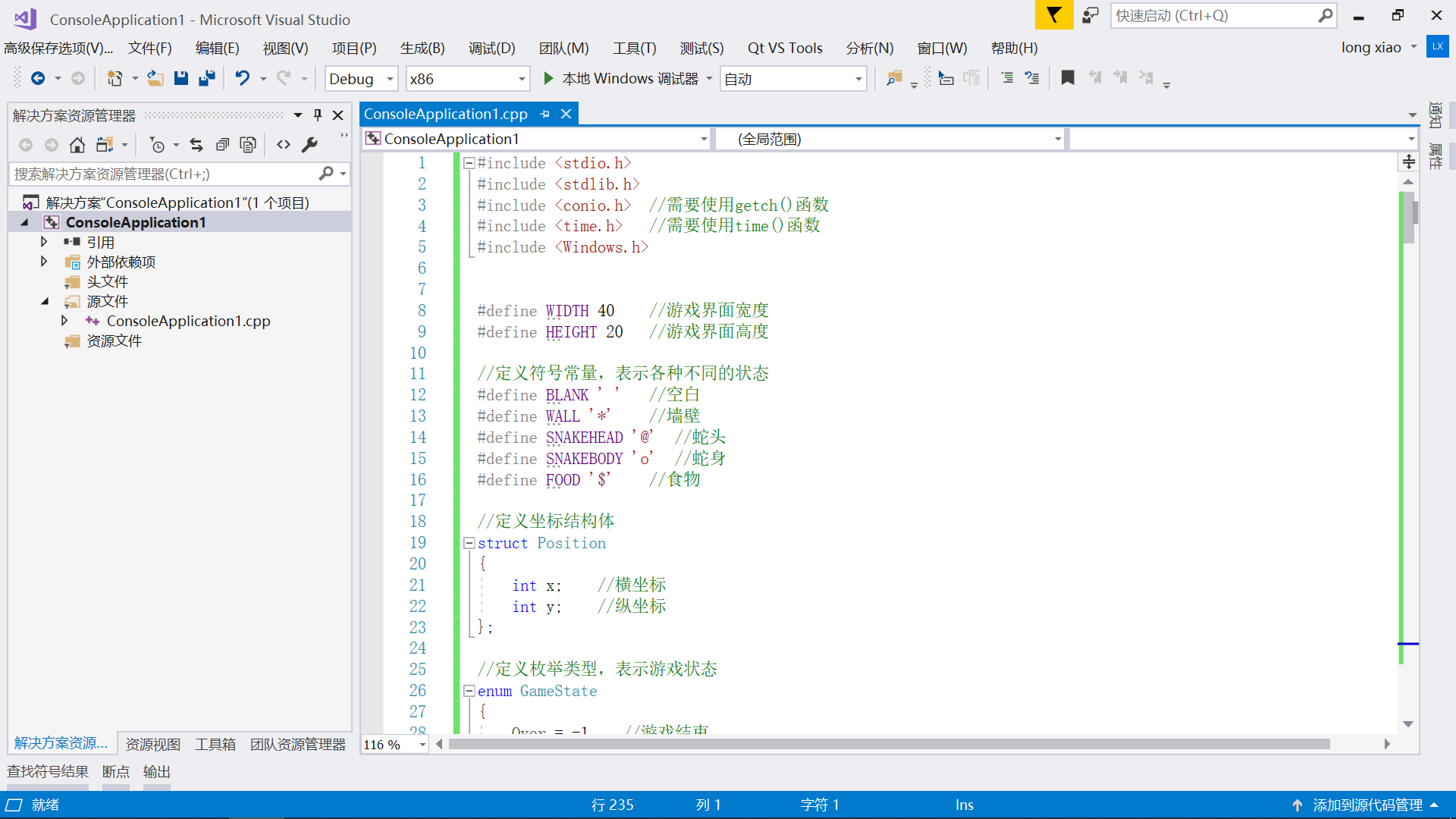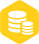# 二、实现效果# 三、完整代码

DrawGame()函数用于绘制游戏画面，将board数组中的字符逐行输出，并在末尾输出得分。UpdateGame()函数用于更新游戏状态，包括蛇的移动、游戏结束判断等。CheckGameOver()函数用于检查游戏是否结束，包括墙壁碰撞、蛇身碰撞、得分达到上限等情况。GenerateFood()函数用于随机生成食物位置，调用rand()函数获取随机数并进行判断。Move()函数用于蛇的移动，根据当前行进方向进行移动。Control()函数用于用户操作，读取键盘输入并更新蛇的行进方向。Pause()函数用于控制游戏速度，通过调用Sleep()函数控制暂停时间。GameOver()函数用于输出最终得分和游戏结束信息，并直接退出程序。``````#include < stdio.h >
#include < stdlib.h >
#include < conio.h >  //需要使用getch()函数
#include < time.h >   //需要使用time()函数
#include < Windows.h >
​
​
#define WIDTH 40    //游戏界面宽度
#define HEIGHT 20   //游戏界面高度
​
//定义符号常量，表示各种不同的状态
#define BLANK ' '   //空白
#define WALL '*'    //墙壁
#define SNAKEBODY 'o'  //蛇身
#define FOOD '\$'    //食物
​
//定义坐标结构体
struct Position
{
int x;    //横坐标
int y;    //纵坐标
};
​
//定义枚举类型，表示游戏状态
enum GameState
{
Over = -1,   //游戏结束
Running = 0, //游戏进行中
Win = 1      //游戏胜利
};
​
int score = 0;   //得分
struct Position body[WIDTH * HEIGHT];  //蛇身位置
struct Position food;   //食物位置
char board[WIDTH][HEIGHT];   //游戏界面
int length = 3;     //蛇身长度，初始为3
int direction = 0;  //蛇的行进方向，0表示向右，1表示向下，2表示向左，3表示向上
​
//函数声明
void InitGame();    //初始化游戏界面和蛇的初始位置
void DrawGame();    //绘制游戏画面
void UpdateGame();  //更新游戏状态
enum GameState CheckGameOver();  //检查游戏是否结束
void GenerateFood();   //生成随机食物
void Move();     //蛇的移动
void Control();  //用户操作，控制蛇的移动
void Pause();    //游戏暂停
void GameOver(); //游戏结束
​
int main()
{
srand(time(NULL));   //用当前时间作为随机数种子，使每次运行的随机食物位置不同
InitGame();   //初始化游戏
DrawGame();   //绘制游戏画面
while (1)
{
UpdateGame();  //更新游戏
DrawGame();    //绘制游戏画面
Control();     //用户操作，控制蛇的移动
Pause();       //游戏暂停
}
return 0;
}
​
//初始化游戏界面和蛇的初始位置
void InitGame()
{
for (int i = 0; i < WIDTH; i++)
{
for (int j = 0; j < HEIGHT; j++)
{
if (i == 0 || j == 0 || i == WIDTH - 1 || j == HEIGHT - 1) //设置墙壁
board[i][j] = WALL;
else
board[i][j] = BLANK;    //其他为空白
}
}
​
//初始化蛇的位置，由一个蛇头和两节身体组成，初始位置在游戏界面的中心
​
board[body.x][body.y] = SNAKEBODY;
​
board[body.x][body.y] = SNAKEBODY;
​
GenerateFood();   //生成随机食物
}
​
//绘制游戏画面
void DrawGame()
{
system("cls");   //清屏，避免前一帧的内容残留
​
for (int i = 0; i < HEIGHT; i++)
{
for (int j = 0; j < WIDTH; j++)
{
printf("%c", board[j][i]);  //输出一个字符
}
printf("\\n");    //每行输出完后，换行
}
​
printf("Score: %d\\n", score);   //输出得分
}
​
//更新游戏状态
void UpdateGame()
{
Move();   //蛇的移动
enum GameState state = CheckGameOver();  //检查游戏是否结束
if (state != Running)  //如果游戏结束，则进行相应操作
{
GameOver();
}
}
​
//检查游戏是否结束
enum GameState CheckGameOver()
{
//蛇头碰到墙壁，游戏结束
return Over;
​
//蛇头碰到蛇身，游戏结束
for (int i = 0; i < length; i++)
{
return Over;
}
​
//吃到食物后，更新分数和蛇的长度，并生成新的食物
{
score += 10;
length++;
GenerateFood();
}
​
//蛇的长度达到游戏界面总格子数减去墙壁的个数，也就是蛇填满游戏界面，游戏胜利
if (length == (WIDTH - 2) * (HEIGHT - 2) - 4)
return Win;
​
return Running;   //游戏继续进行
}
​
//生成随机食物
void GenerateFood()
{
int x, y;
do
{
x = rand() % (WIDTH - 2) + 1;    //随机x坐标，排除在边框上的墙壁位置
y = rand() % (HEIGHT - 2) + 1;   //随机y坐标，排除在边框上的墙壁位置
} while (board[x][y] != BLANK);   //如果随机到的位置不为空白，则重新随机
food.x = x;
food.y = y;
board[x][y] = FOOD;  //在随机位置生成食物
}
​
//蛇的移动
void Move()
{
//更新蛇身的位置，从后往前移动
for (int i = length - 1; i > 0; i--)
{
body[i].x = body[i - 1].x;
body[i].y = body[i - 1].y;
board[body[i].x][body[i].y] = SNAKEBODY;
}
​
//更新蛇头的位置
switch (direction)   //根据蛇头行进方向进行移动
{
case 0:  //向右
break;
case 1:  //向下
break;
case 2:  //向左
break;
case 3:  //向上
break;
}
board[body[length - 1].x][body[length - 1].y] = BLANK;  //清除蛇尾
}
​
//用户操作，控制蛇的移动
void Control()
{
if (kbhit())   //如果有按键按下
{
char ch = getch();   //获取按键字符
switch (ch)
{
case 'w':
if (direction != 1)  //避免蛇头掉头
direction = 3;
break;
case 's':
if (direction != 3)
direction = 1;
break;
case 'a':
if (direction != 0)
direction = 2;
break;
case 'd':
if (direction != 2)
direction = 0;
break;
case 'q':
GameOver();  //按下'q'键退出游戏
break;
}
}
}
​
//游戏暂停
void Pause()
{
Sleep(150);   //每次循环暂停一段时间，控制蛇的移动速度
}
​
//游戏结束
void GameOver()
{
system("cls");   //清屏，输出最终得分和游戏结束信息
printf("Game over!\\n");
exit(0);  //直接退出程序
}
``````×
20